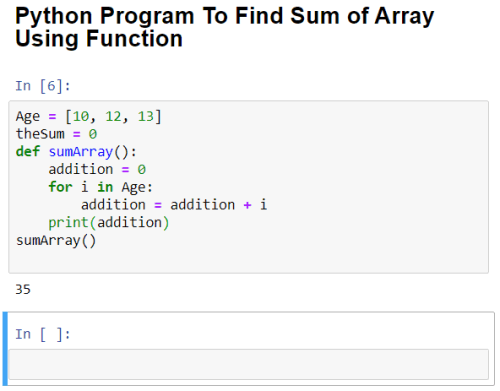# Python 3 Program To Find The Sum of An Array

3568In this tutorial, you will learn a simple Python program to find the sum of an array. To understand this Python program, you should have an understanding of the following concepts:

We can do this by 2 methods. First, we can calculate the sum of all the elements in the array without using functions. Secondly, we can create a function to add all the elements of an array and then print the sum by calling that function.

Recommended Read – 10 Best Books To Learn Python For Beginners And Experts.

Let’s see how we can do this by these 2 methods.

## Python 3 Program To Find Sum of Array Without Function

Source Code:

```Age = [10, 12, 13]       #Inputting Array values.
theSum = 0               #Definig variable to store the sum of array.
for i in Age:            #For loop to iterate through the array
theSum = theSum + i     #Adding array elements.

print(theSum)            #Outputting the sum of array elements.```

## OutputRelated postHow To Use ArcGIS API for Python and Jupyter Notebooks.

## Python 3 Program To Find Sum of Array Using Function

Source code:

```Age = [10, 12, 13]
def sumArray():